How to Use a Multimeter From National Instruments

Publish Date: Feb 03, 2017 | 55 Ratings | 3.09 out of 5 | Print

Overview

The digital multimeter is one of the most versatile electrical instruments, capable of acquiring a variety of measurements. Digital multimeters are easy to use and are a necessity when testing and debugging electronics or electrical circuits. Digital multimeters have multiple functionality and serve as a voltmeter, ammeter, and ohmmeter combined in one package. Hand-held digital multimeters are sufficient for simple electrical measurements but for increased speed, accuracy, and functionality more robust digital multimeters are needed, such as the NI 4070 FlexDMM. The NI 4070 has 6½ digits of precision and also has the functionality of a fully isolated, high-voltage digitizer, capable of acquiring waveforms at sampling rates up to 1.8 MS/s at ±300 V input. This document describes the common measurements that can be made with digital multimeters as well as advanced features of the NI 4070 Digital Multimeter.

1. Voltage Measurements

A digital multimeter can be used as a voltmeter to determine the potential voltage difference across several leads of an electrical component or from a lead referenced to ground. To measure the voltage across two leads, place the positive terminal on the lead with higher voltage (if known) and the negative terminal on the lead with a lower voltage. To measure the voltage of a specific location referenced to ground, connect the positive terminal to the desired location and the negative terminal to ground. When used as a voltmeter, the digital multimeter has a very large input impedance and thus draws very little current. Many digital multimeters, such as the NI 4070, are capable of testing both AC and DC voltages. The figures below show the connections needed to measure an AC or DC voltage signal with an NI 4070. For more information about voltage measurements and some of the common factors that affect voltage measurements, refer to the Digital Multimeters.

 DC Voltage Measurement AC Voltage Measurement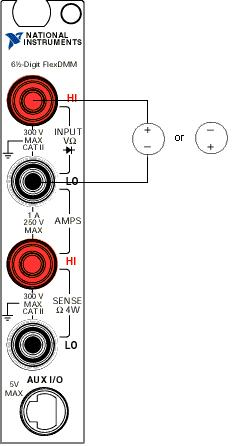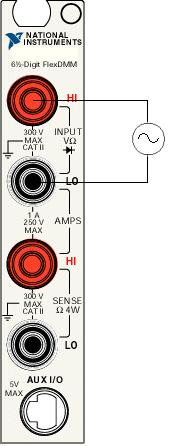2. Current Measurements

A digital multimeter can be used as an ammeter to determine the current flow through a wire or electrical component. This measurement is accomplished by placing the digital multimeter in series with the wire that the current is flowing through. When used as an ammeter, the digital multimeter has a very small impedance (resistance) resulting in a small voltage drop across the multimeters leads. Many digital multimeters, such as the NI 4070, are capable of testing both AC and DC currents. The figure below shows the connections needed to make a current measurement with an NI 4070. For more information about current measurements and some of the common factors that affect current measurements, refer to the Digital Multimeters.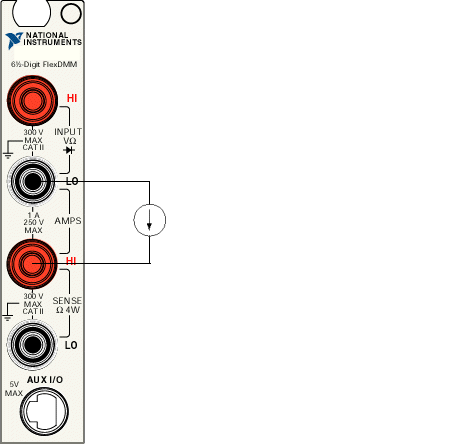3. Resistance Measurements

A digital multimeter can be used as an ohmmeter to measure resistance of an electrical component. This measurement is accomplished by placing the digital multimeter's terminals across the electrical component where a resistance measurement is to be taken. When switched to the ohmmeter setting the digital multimeter develops a small known voltage between its terminals. When a resistor is connected across these terminals a current will flow. The digital multimeter then measures this current and the resistance is calculated using Ohm's Law (V = I * R). Many digital multimeters, such as the NI 4070, are capable of making 2-wire and 4-wire resistance measurements. The figures below show the connections needed to make resistance measurements with an NI 4070. For more information about resistance measurements and some of the common factors that affect resistance measurements, refer to the Digital Multimeters.

 2-Wire Resistance Measurement 4-Wire Resistance Measurement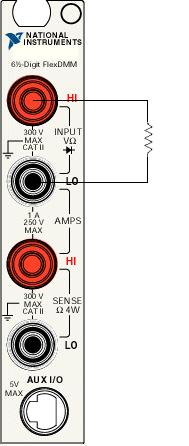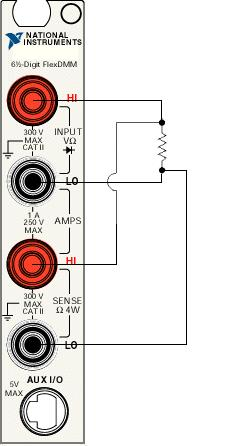4. Continuity Measurements

A digital multimeter can be used to measure continuity between two points of a circuit. This is a great test to determine if circuits are continuous, thereby allowing current to flow. Some digital multimeters, such as the NI 4070, have a continuity setting to determine if current can flow between two points. A continuity test can still be accomplished even if the multimeter does not have a continuity setting though. By using a resistance measurement, a user can determine if there is an open circuit (infinite resistance) or closed circuit (low resistance) between two points. The figure below shows the connections needed to make a continuity measurement with an NI 4070. For more information about continuity measurements and some of the common factors that affect continuity measurements, refer to the Digital Multimeters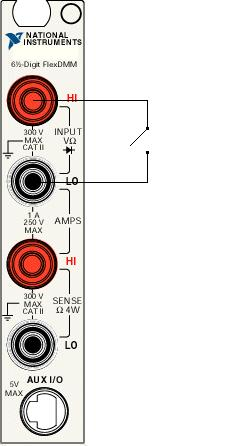5. Frequency Measurements

Some digital multimeters, such as the NI 4070, are capable of making accurate frequency measurements. Maintaining the right frequency is crucial for electrical devices that rely on a steady AC voltage and current. Frequency is measured in hertz (Hz), the number of times per second a waveform repeats, and can be accomplished by attaching the positive terminal to the electrical component with the signal and the negative lead to ground. The figure below shows the connections needed to make a frequency measurement with an NI 4070. For more information about frequency measurements and some of the common factors that affect frequency measurements, refer to the Digital Multimeters.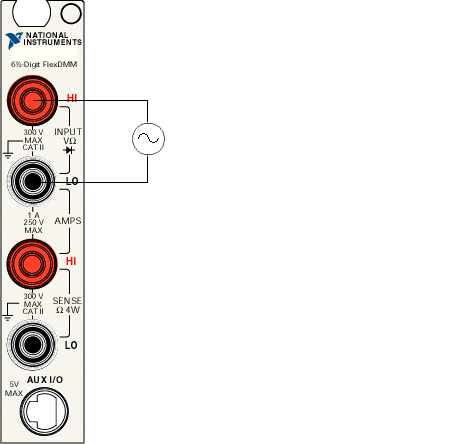6. Diode Measurements

Diode measurement and testing is critical for the semiconductor and telecommunications industries. Because of this demand a select number of digital multimeters, such as the NI 4070, have a mode that easily allows the user to make diode measurements. The process for taking a diode measurement involves supplying the diode with a constant current source and reading the resulting voltage drop across the leads. The figure below shows the connections needed to make a diode measurement with an NI 4070. For more information about diode measurements refer to the Digital Multimeters.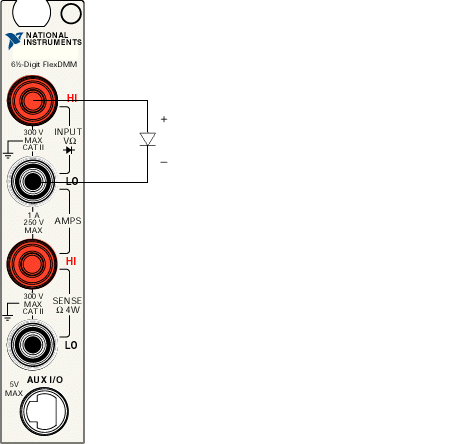In addition to the common digital multimeter measurements described above, the NI 4070 Digital Multimeter is capable of performing many advanced measurements. The NI 4070 is able to take application specific power measurements such as real power, the measure of a circuit's dissipative elements (R); reactive power, the measure of a circuit's reactance (X); and apparent power, the measure of a circuit's impedance (Z). Several other unique measurements the NI 4070 is capable of are (1) acquiring the noise on a power supply output and (2) acquisition of flyback voltages.

A characterisic that is unique to the NI 4070 is its capability to operate as a fully isolated, high-voltage digitizer, capable of acquiring waveforms at sample rates up to 1.8 MS/s at ±300 V input. As an isolated digitizer a user can measure differential waveforms with high levels of common-mode voltage and vary the resolution from 10 to 23 bits. For more information about digitizer measurements refer to the Digital Multimeters.

Ratings

Rate this document